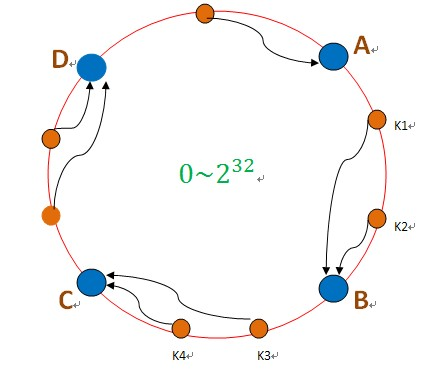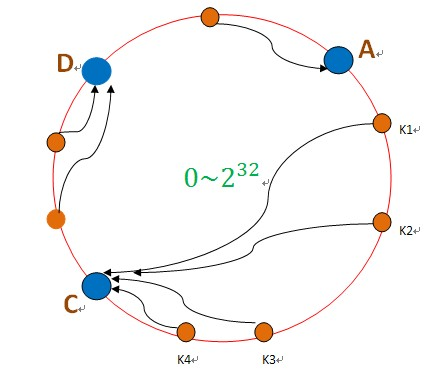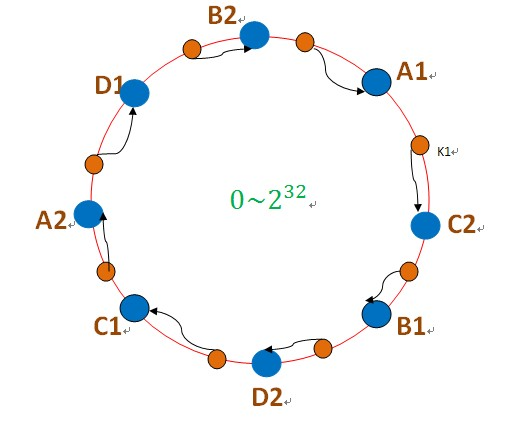# 一致性哈希算法与C++实现

## 一. 算法解决问题

1、平衡性(Balance)：平衡性是指哈希的结果能够尽可能分布到所有的缓冲中去，这样可以使得所有的缓冲空间都得到利用。很多哈希算法都能够满足这一条件。
2、单调性(Monotonicity)：单调性是指如果已经有一些内容通过哈希分派到了相应的缓冲中，又有新的缓冲加入到系统中。哈希的结果应能够保证原有已分配的内容可以被映射到原有的或者新的缓冲中去，而不会被映射到旧的缓冲集合中的其他缓冲区。
3、分散性(Spread)：在分布式环境中，终端有可能看不到所有的缓冲，而是只能看到其中的一部分。当终端希望通过哈希过程将内容映射到缓冲上时，由于不同终端所见的缓冲范围有可能不同，从而导致哈希的结果不一致，最终的结果是相同的内容被不同的终端映射到不同的缓冲区中。这种情况显然是应该避免的，因为它导致相同内容被存储到不同缓冲中去，降低了系统存储的效率。分散性的定义就是上述情况发生的严重程度。好的哈希算法应能够尽量避免不一致的情况发生，也就是尽量降低分散性。
4、负载(Load)：负载问题实际上是从另一个角度看待分散性问题。既然不同的终端可能将相同的内容映射到不同的缓冲区中，那么对于一个特定的缓冲区而言，也可能被不同的用户映射为不同 的内容。与分散性一样，这种情况也是应当避免的，因此好的哈希算法应能够尽量降低缓冲的负荷。

## 二. 设计方法## 三. 代码实现

MurMurHash算法，是非加密HASH算法，性能很高， 比传统的CRC32, MD5，SHA-1（这两个算法都是加密HASH算法，复杂度本身就很高，带来的性能上的损害也不可避免） 等HASH算法要快很多，而且这个算法的碰撞率很低。所以这里我们采用MurMurHash算法

#include <map>
using namespace std;

class ConsistentHash
{
public:
ConsistentHash(int node_num, int virtual_node_num);
~ConsistentHash();

void Initialize();
size_t GetServerIndex(const char* key);

void DeleteNode(const int index);
void AddNewNode(const int index);

private:
map<uint32_t,size_t> server_nodes_; //虚拟节点,key是哈希值，value是机器的index
int node_num_;//真实机器节点个数
int virtual_node_num_;//每个机器节点关联的虚拟节点个数
};

#include <map>
#include <string.h>
#include <sstream>
#include "consistent_hash.h"
#include "murmurhash3.h"
using namespace std;

ConsistentHash::ConsistentHash(int node_num, int virtual_node_num)
{
node_num_ = node_num;
virtual_node_num_ = virtual_node_num;
}

ConsistentHash::~ConsistentHash()
{
server_nodes_.clear();
}

void ConsistentHash::Initialize()
{
for(int i=0; i<node_num_; ++i)
{
for(int j=0; j<virtual_node_num_; ++j)
{
stringstream node_key;
node_key<<"SHARD-"<<i<<"-NODE-"<<j;
uint32_t partition = murmur3_32(node_key.str().c_str(), strlen(node_key.str().c_str()));
server_nodes_.insert(pair<uint32_t, size_t>(partition, i));
}
}
}

size_t ConsistentHash::GetServerIndex(const char* key)
{
uint32_t partition = murmur3_32(key, strlen(key));
map<uint32_t, size_t>::iterator it = server_nodes_.lower_bound(partition);//沿环的顺时针找到一个大于等于key的虚拟节点

if(it == server_nodes_.end())//未找到
{
return server_nodes_.begin()->second;
}
return it->second;
}

void ConsistentHash::DeleteNode(const int index)
{
for(int j=0; j<virtual_node_num_; ++j)
{
stringstream node_key;
node_key<<"SHARD-"<<index<<"-NODE-"<<j;
uint32_t partition = murmur3_32(node_key.str().c_str(), strlen(node_key.str().c_str()));
map<uint32_t,size_t>::iterator it = server_nodes_.find(partition);
if(it != server_nodes_.end())
{
server_nodes_.erase(it);
}
}
}

void ConsistentHash::AddNewNode(const int index)
{
for(int j=0; j<virtual_node_num_; ++j)
{
stringstream node_key;
node_key<<"SHARD-"<<index<<"-NODE-"<<j;
uint32_t partition = murmur3_32(node_key.str().c_str(), strlen(node_key.str().c_str()));
server_nodes_.insert(pair<uint32_t, size_t>(partition, index));
}
}

## 四. 测试结果

consistent hash initialize success, node_num=5, virtual_num=100
key = 4, index = 3
key = 7, index = 3
key = 0, index = 3
key = 1, index = 3
key = 6, index = 3
key = 3, index = 3
key = 5, index = 0
key = 2, index = 4
key = 8, index = 2
key = 9, index = 0
node error,index = 3
key = 3, index = 4
key = 7, index = 2
key = 4, index = 1
key = 0, index = 2
key = 1, index = 1
key = 6, index = 4
node recover,index = 3
key = 4, index = 3
key = 3, index = 3
key = 0, index = 3
key = 1, index = 3
key = 7, index = 3
key = 6, index = 3
index = 0, data_count = 5985
index = 1, data_count = 1991
index = 2, data_count = 5041
index = 3, data_count = 12014
index = 4, data_count = 4969